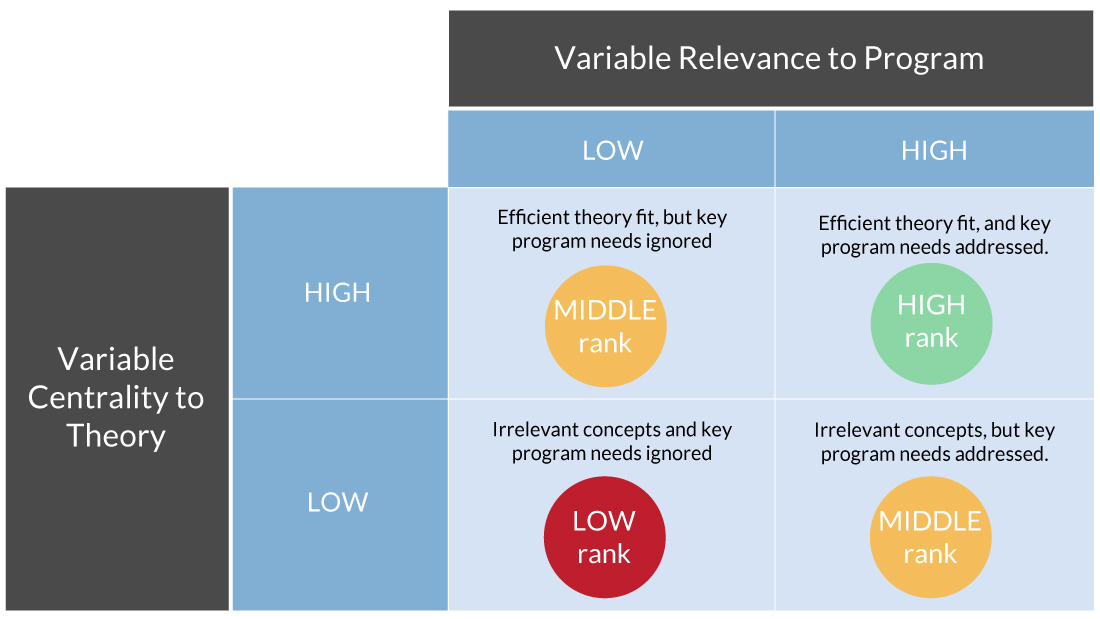# Behind the Curtain

## Ranking Scheme

The alpha version of TheoryPicker calculates and sorts scores for each of 6 theories. See a conceptual version of the sorting algorithm below.## Theory score calculation:

1. A user of the tool answers 18 non-technical questions about a particular behavior change objective. Responses are coded 1-3. They indicate the relevance of each of 18 variable categories to the user’s behavior of interest.
2. Centrality of a variable category to a theory was determined three times to reflect theory evolution, producing 3 variable category x theory matrices . They allow the user to select one of three modes for ranking the theories. Original mode assigns the value 3 to a theory’s original constructs and 1 to all others; Interim mode assigns 3 to original constructs, 2 to subsequently added constructs, and 1 to the others; and Current mode assigns 3 to every construct in a recent version of a theory. The point of reference was Theory at a Glance, 2nd ed., or Glanz et al., (2008).1
3. Relevance and centrality ratings from steps A & B are summed for each construct, and the construct sums are summed within each theory.
4. Each theory-wise sum is divided by the number of variables in the theory to remove bias introduced when a theory has a relatively large number of constructs.
5. Quotients generated in step D are sorted from highest to lowest numerical value. Then:
1. If acquiring skill was rated as irrelevant to the behavior in step B, SCT is ranked last. This is done because building skills can demand substantial program resources.
2. If a behavior must be performed only once, TTM is moved to last place because it includes stages in which behaviors are maintained over time.
3. Ties are allowed and labeled as such.

## Results

Results are being validated against findings of published research that compared the relative effectiveness of two or more of the theories empirically.5